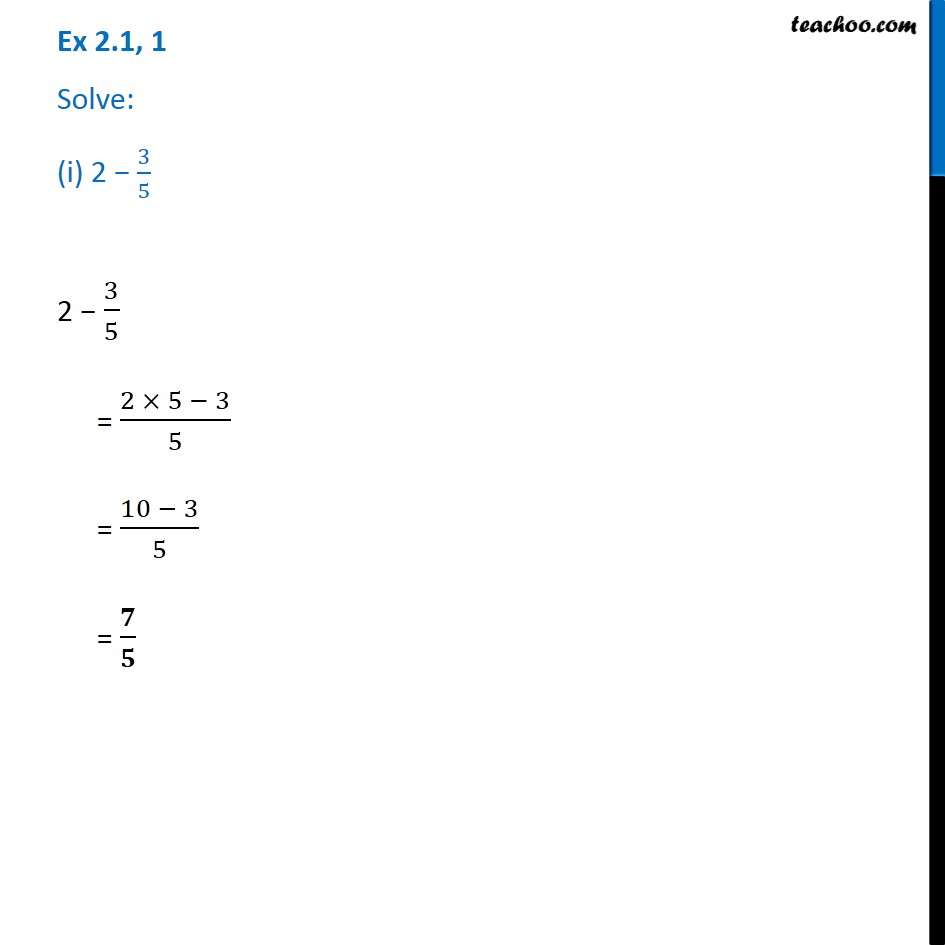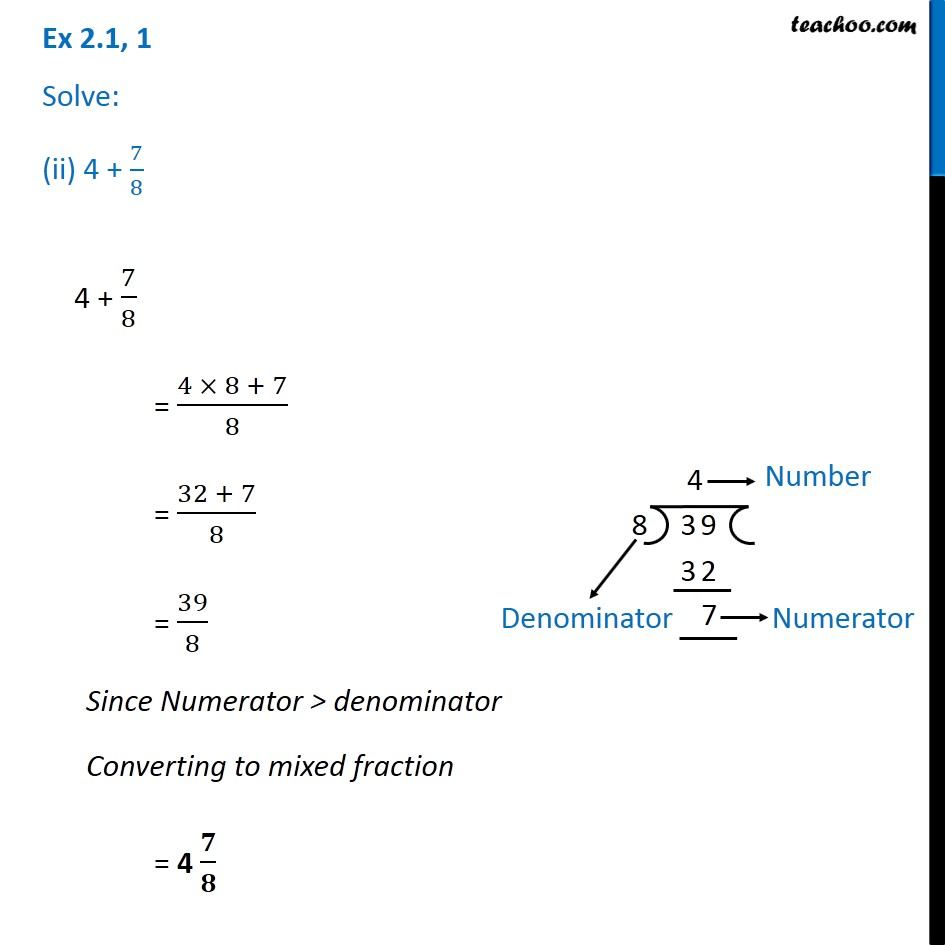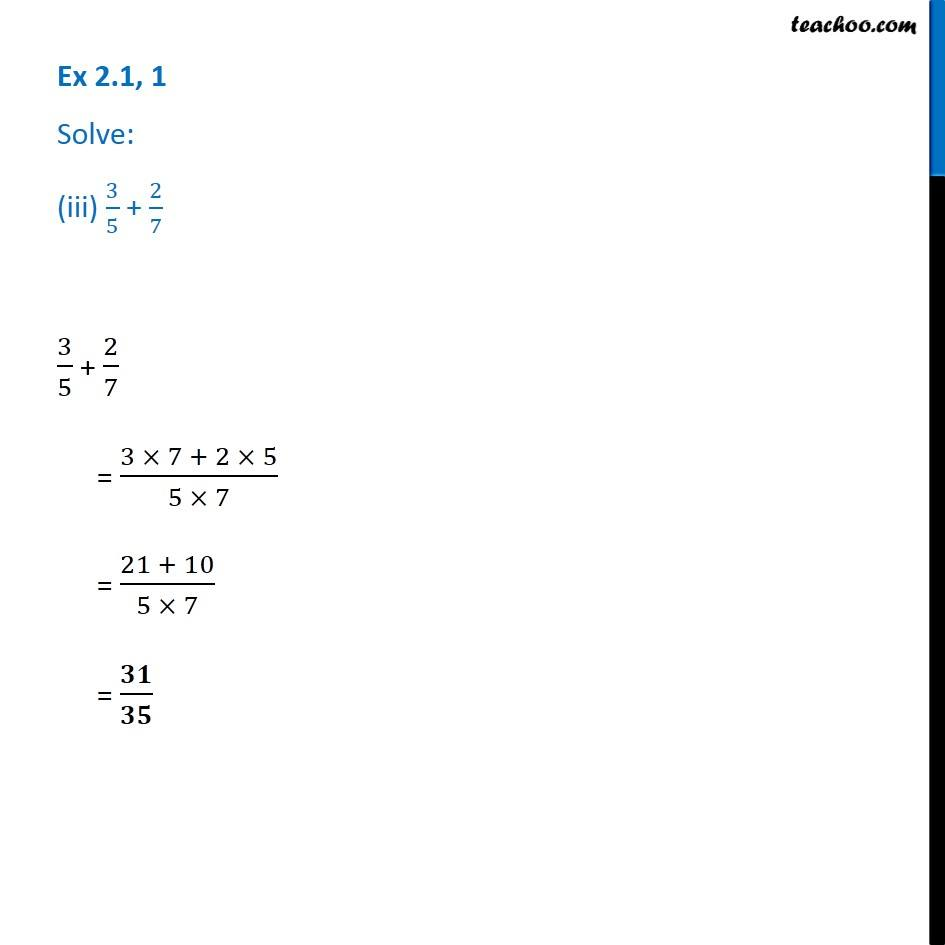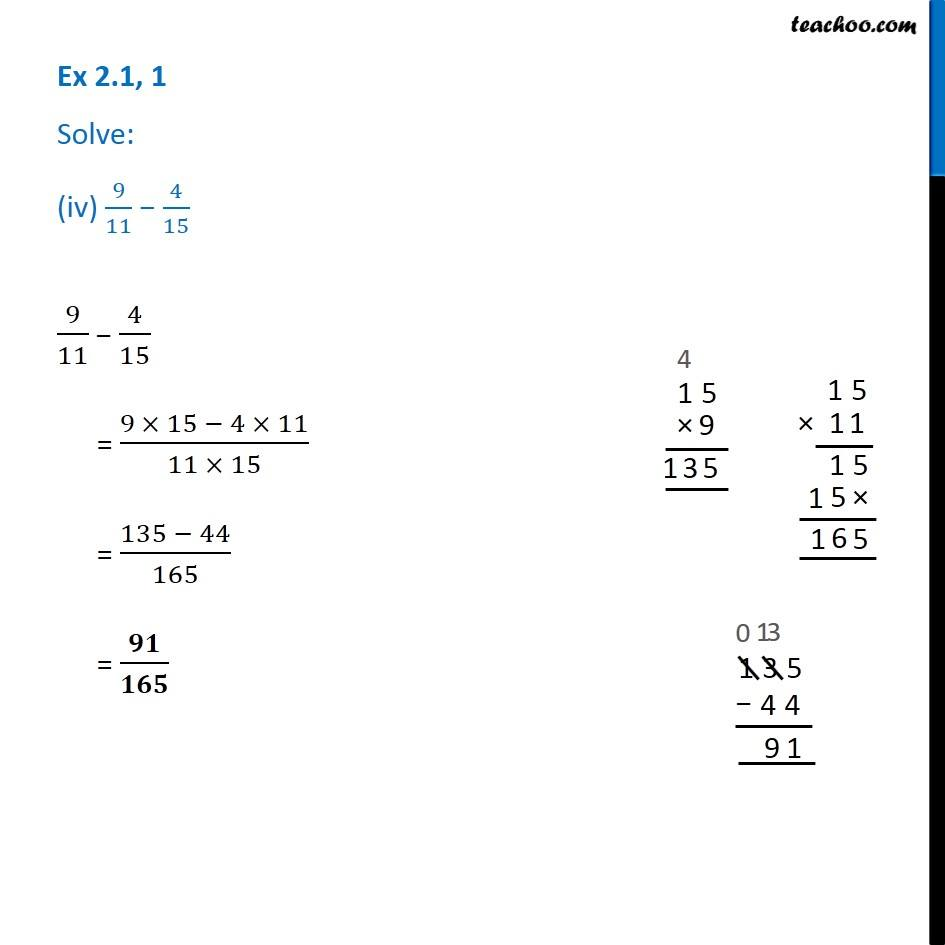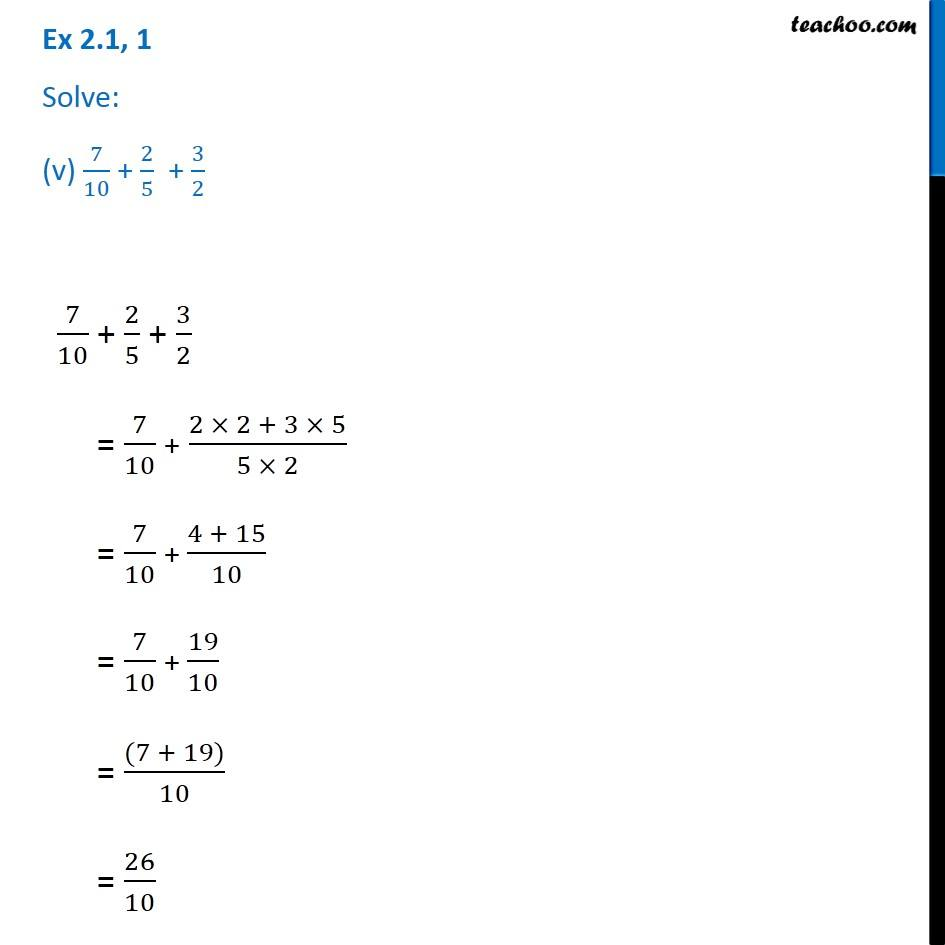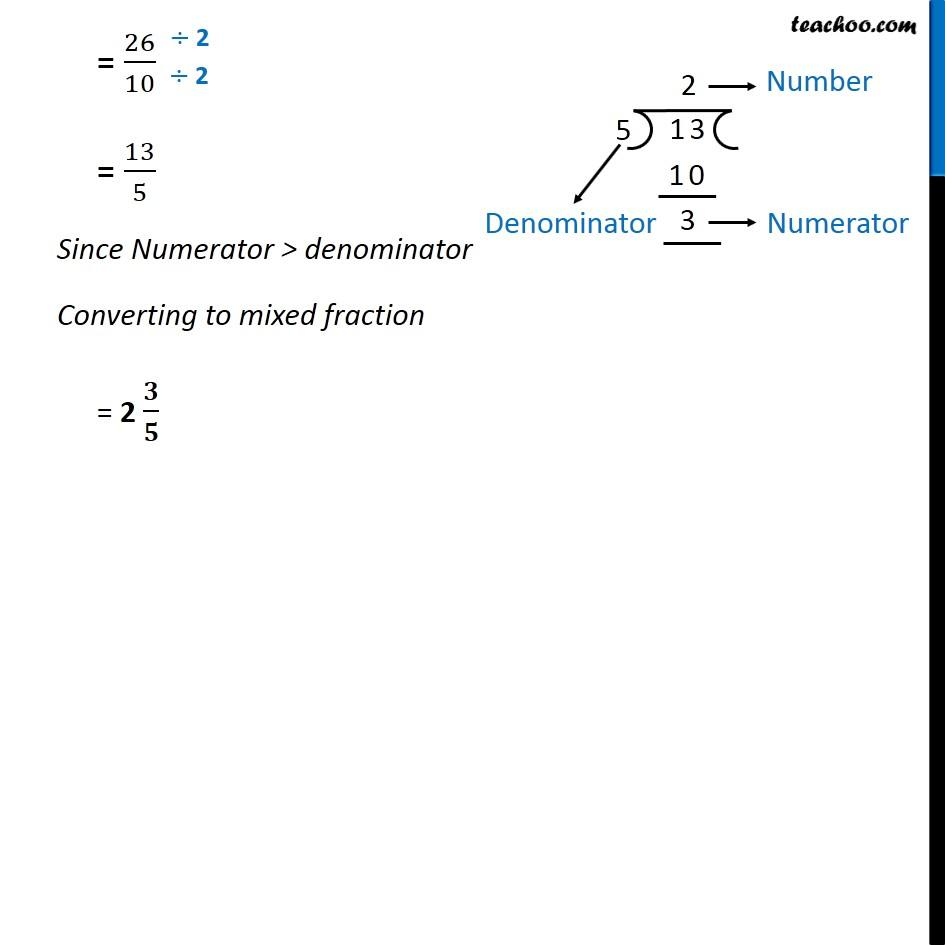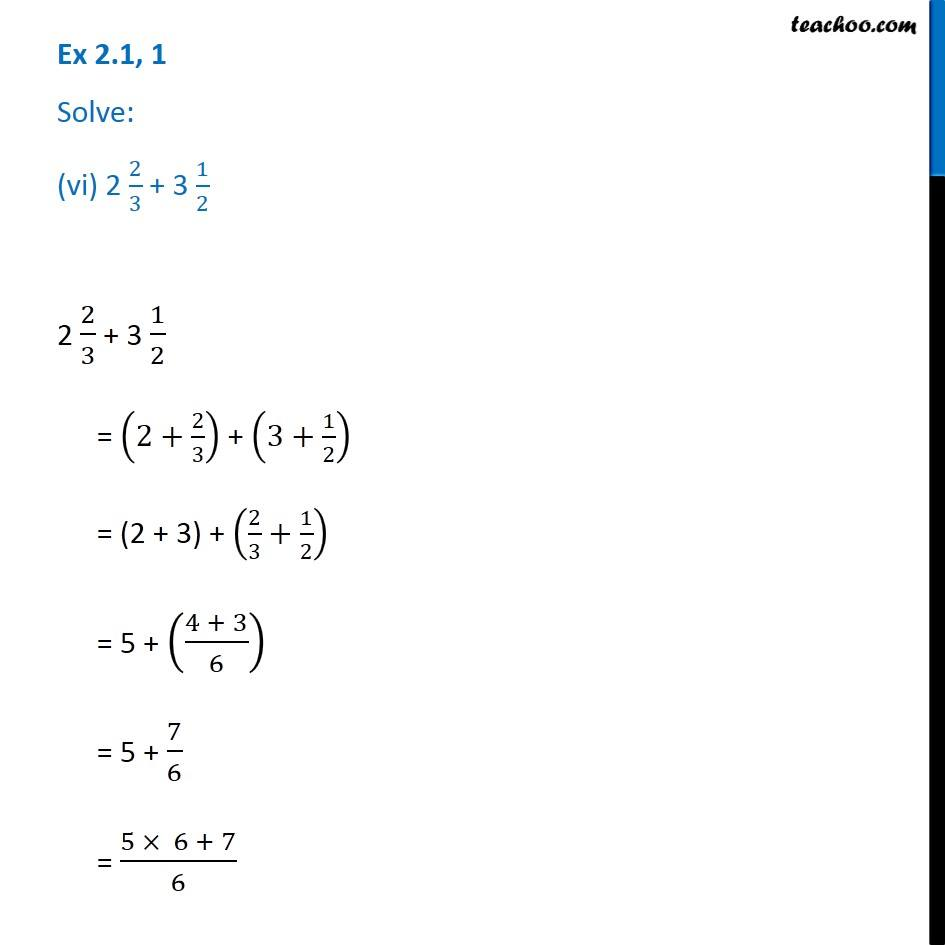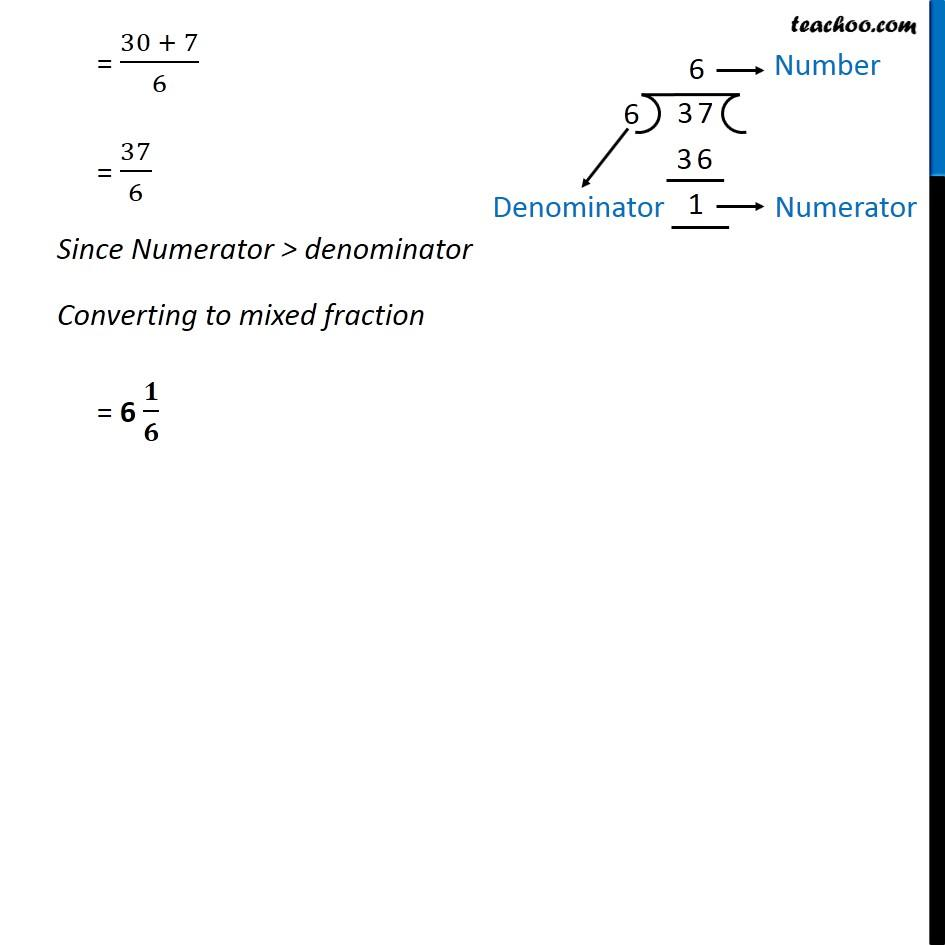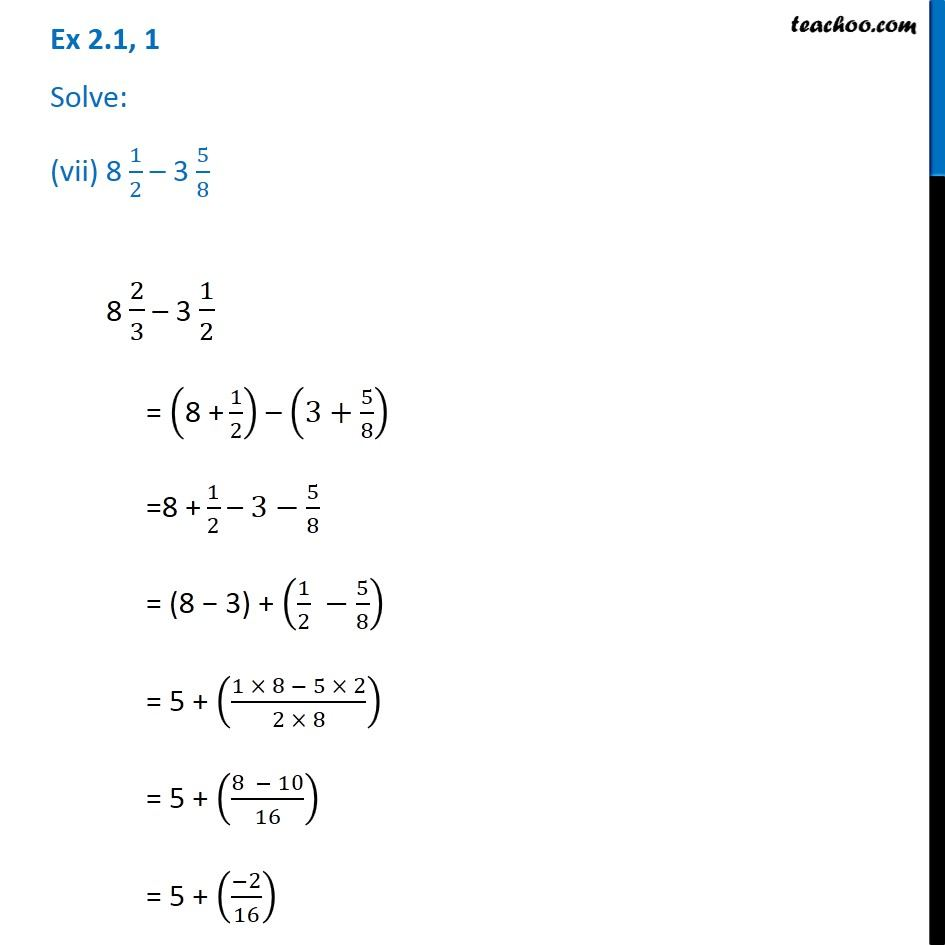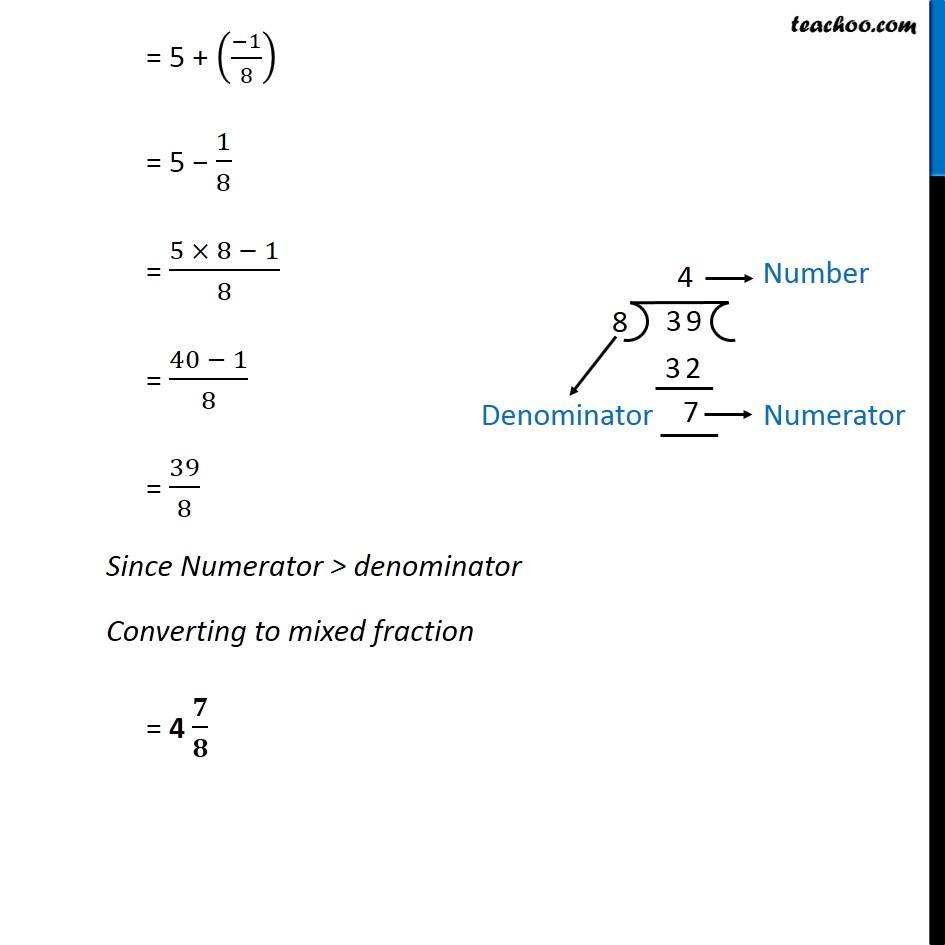Subscribe to our Youtube Channel - https://you.tube/teachoo

1. Chapter 2 Class 7 Fractions and Decimals
2. Serial order wise
3. Ex 2.1

Transcript

Ex 2.1, 1 Solve: (i) 2 − 3/5 2 − 3/5 = (2 × 5 − 3)/5 = (10 − 3)/5 = 𝟕/𝟓 Ex 2.1, 1 Solve: (ii) 4 + 7/8 4 + 7/8 = (4 × 8 + 7)/8 = (32 + 7)/8 = 39/8 Since Numerator > denominator Converting to mixed fraction = 4 𝟕/𝟖 Ex 2.1, 1 Solve: (iii) 3/5 + 2/7 3/5 + 2/7 = (3 × 7 + 2 × 5)/(5 × 7) = (21 + 10)/(5 × 7) = 𝟑𝟏/𝟑𝟓 Ex 2.1, 1 Solve: (iv) 9/11 − 4/15 9/11 − 4/15 = (9 × 15 − 4 × 11)/(11 × 15) = (135 − 44)/165 = 𝟗𝟏/𝟏𝟔𝟓 Ex 2.1, 1 Solve: (v) 7/10 + 2/5 + 3/27/10 + 2/5 + 3/2 = 7/10 + (2 × 2 + 3 × 5)/(5 × 2) = 7/10 + (4 + 15)/10 = 7/10 + 19/10 = ((7 + 19))/10 = 26/10 = 26/10 = 13/5 Since Numerator > denominator Converting to mixed fraction = 2 𝟑/𝟓 Ex 2.1, 1 Solve: (vi) 2 2/3 + 3 1/2 2 2/3 + 3 1/2 = (2+2/3) + (3+1/2) = (2 + 3) + (2/3+1/2) = 5 + ((4 + 3)/6) = 5 + 7/6 = (5 × 6 + 7)/6 = (30 + 7)/6 = 37/6 Since Numerator > denominator Converting to mixed fraction = 6 𝟏/𝟔 Ex 2.1, 1 Solve: (vii) 8 1/2 – 3 5/8 8 2/3 – 3 1/2 = ("8 +" 1/2) – (3+5/8) ="8 +" 1/2 – 3−5/8 = (8 − 3) + (1/2 −5/8) = 5 + ((1 × 8 − 5 × 2)/(2 × 8)) = 5 + ((8 − 10)/16) = 5 + ((−2)/16) = 5 + ((−1)/8) = 5 − 1/8 = (5 × 8 − 1)/8 = (40 − 1)/8 = 39/8 Since Numerator > denominator Converting to mixed fraction = 4 𝟕/𝟖

Ex 2.1